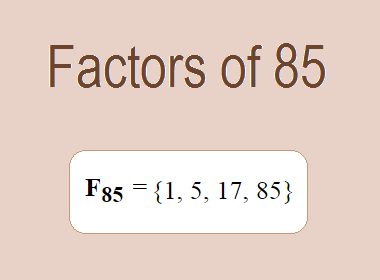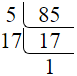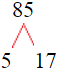# Factors of 85The factors of 85 are 1, 5, 17, and 85 i.e. F85 = {1, 5, 17, 85}. The factors of 85 are all the numbers that can divide 85 without leaving a remainder.

We can check if these numbers are factors of 85 by dividing 85 by each of them. If the result is a whole number, then the number is a factor of 85. Let's do this for each of the numbers listed above:

·        1 is a factor of 85 because 85 divided by 1 is 85.

·        5 is a factor of 85 because 85 divided by 5 is 17.

·        17 is a factor of 85 because 85 divided by 17 is 5.

·        85 is a factor of 85 because 85 divided by 85 is 1.

## How to Find Factors of 85?

1 and the number itself are the factors of every number. So, 1 and 85 are two factors of 85. To find the other factors of 85, we can start by dividing 85 by the numbers between 1 and 85. If we divide 85 by 2, we get a remainder of 1. Therefore, 2 is not a factor of 85. If we divide 85 by 3, we get a remainder of 1. Therefore, 3 is not a factor of 85.

Next, we can check if 4 is a factor of 85. If we divide 85 by 4, we get a remainder of 1. Therefore, 4 is also not a factor of 85. If we divide 85 by 5, we get a remainder of 0. Therefore, 5 is a factor of 85. We can continue this process for all the possible factors of 85.

Through this process, we can find that the factors of 85 are 1, 5, 17, and 85. These are the only numbers that can divide 85 without leaving a remainder.

********************

********************

## Properties of the Factors of 85

The factors of 85 have some interesting properties. One of the properties is that the sum of the factors of 85 is equal to 108. We can see this by adding all the factors of 85 together:

1 + 5 + 17 + 85 = 108

Another property of the factors of 85 is that they are all odd numbers. This is because 85 is an odd number, and any even number cannot divide an odd number without leaving a remainder.

Another property of the factors of 85 is that the prime factors of 85 are 5 and 17.

## Applications of the Factors of 85

The factors of 85 have several applications in mathematics. One of the applications is in finding the highest common factor (HCF) of two or more numbers. The HCF is the largest factor that two or more numbers have in common. For example, to find the HCF of 85 and 34, we need to find the factors of both numbers and identify the largest factor they have in common. The factors of 85 are 1, 5, 17, and 85. The factors of 34 are 1, 2, 17, and 34. The largest factor that they have in common is 17. Therefore, the HCF of 85 and 34 is 17.

Another application of the factors of 85 is in prime factorization. Prime factorization is the process of expressing a number as the product of its prime factors. The prime factors of 85 are 5 and 17. We can express 85 as:

85 = 5 × 17

We can do prime factorization by division and factor tree method also. Here is the prime factorization of 85 by division method,85 = 5 × 17

Here is the prime factorization of 85 by the factor tree method,85 = 5 × 17

## Conclusion

The factors of 85 are the numbers that can divide 85 without leaving a remainder. The factors of 85 are 1, 5, 17, and 85. The factors of 85 have some interesting properties, such as being odd numbers and having a sum of 108. The factors of 85 have several applications in mathematics, such as finding the highest common factor and prime factorization.